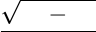Study Guides (390,000)
US (220,000)
UC-Irvine (3,000)
MATH (600)
MATH 2B (100)
All (60)
Final

# MATH 2B Study Guide - Final Guide: Trihydrogen Cation, 4DxExam

Department
Mathematics
Course Code
MATH 2B
Professor
All
Study Guide
Final

This preview shows pages 1-2. to view the full 8 pages of the document.MATH 2B: SAMPLE FINAL #1
This exam consists of 14 questions and 100 total points.
Read the directions for each problem carefully and answer all parts of each problem.
Please show all work needed to arrive at your solutions (unless instructed otherwise). Label
graphs and deﬁne any notation used. Cross out incorrect scratch-work.
No calculators or other forms of assistance are allowed. Do not check your cell phones
during the exam.
1. (6 points) Suppose that R1
1f(x)dx = 6,R4
1f(x)dx =2and R1
1h(x)dx = 9. Use this
information to compute the following.
a. R1
46f(x)dx
b. R1
12f(x) + 3h(x)dx
c. R4
1f(x)dx
2. (6 points)
a. Evaluate the following derivative
d
dx Zx2
sin(x)
t3tan(t)dt.
b. Let r(t)be the rate at which the world’s oil is consumed, where tis measured in years starting
at t= 0 representing January 1, 2000, and r(t)is measured in barrels per year. What does
R13
0r(t)dt represent and what are its units?

Only pages 1-2 are available for preview. Some parts have been intentionally blurred.3. (6 points) Evaluate Rx2tan1x dx
4. (6 points) Evaluate Z1
xln(3x)dx

Unlock to view full version

Only pages 1-2 are available for preview. Some parts have been intentionally blurred.5. (6 points) Evaluate Rsin5(x) cos2(x)dx
6. (6 points) Evaluate Zx225
xdx, where x > 5